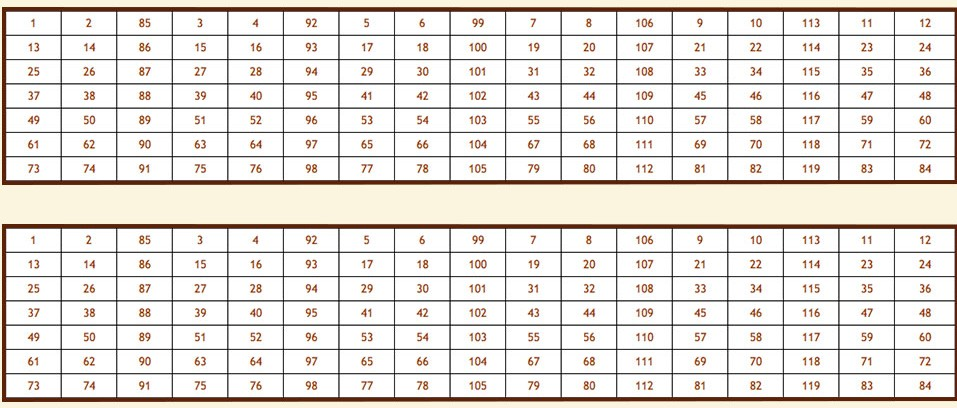# St. Joseph's Cemetery Register

Surname: Year of Death:

## Section 1: plot numbers## Section 2: plot numbers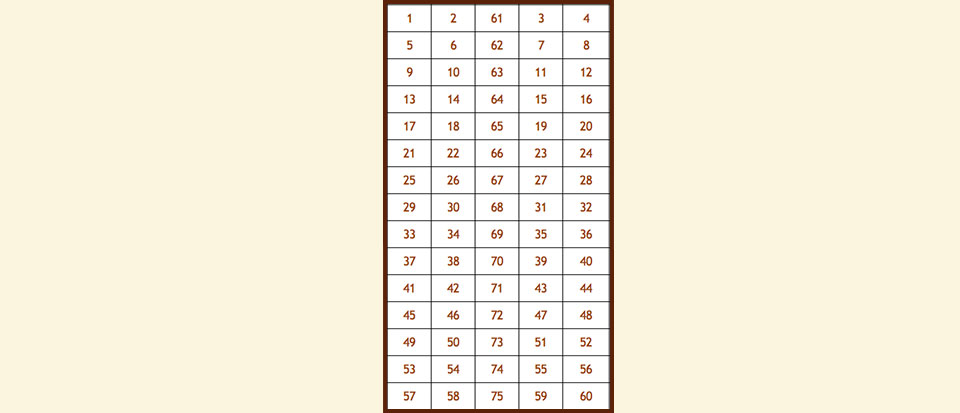## Section 3: plot numbers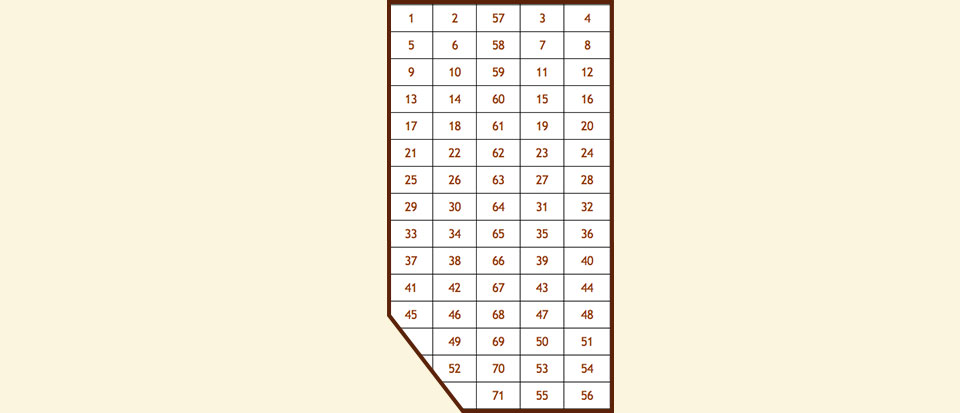## Section 4: plot numbers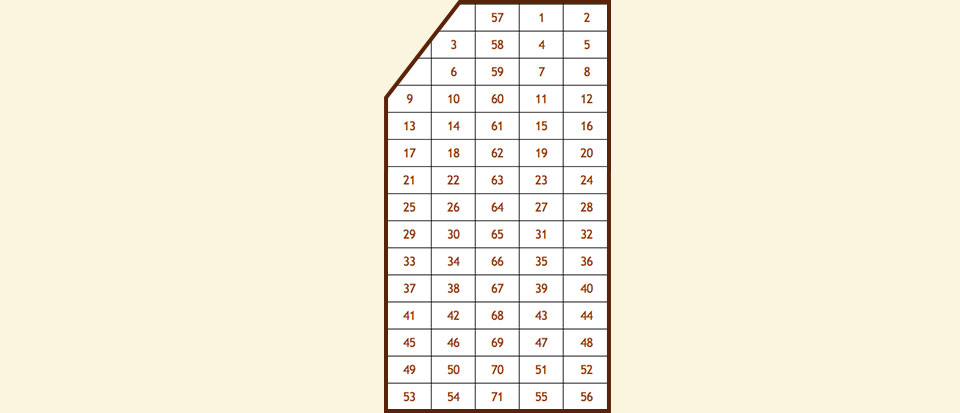## Section 5: plot numbers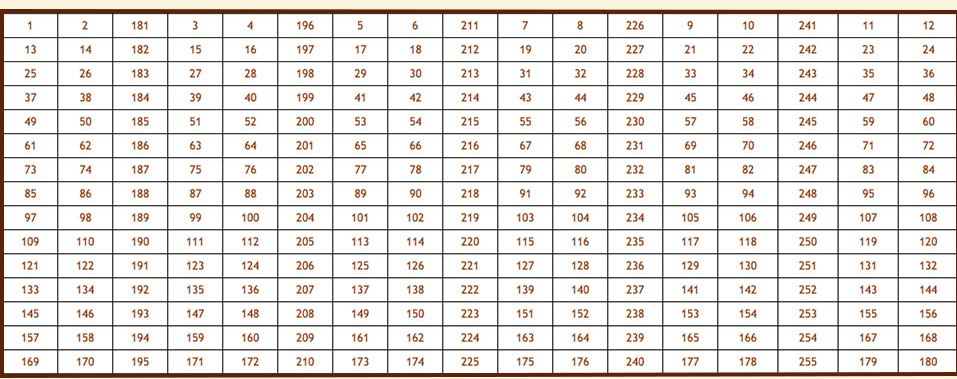## Section 6: plot numbers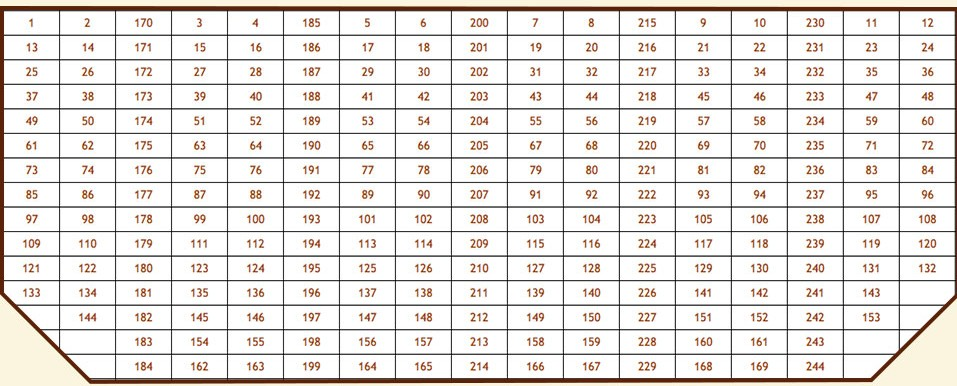## Section 7: plot numbers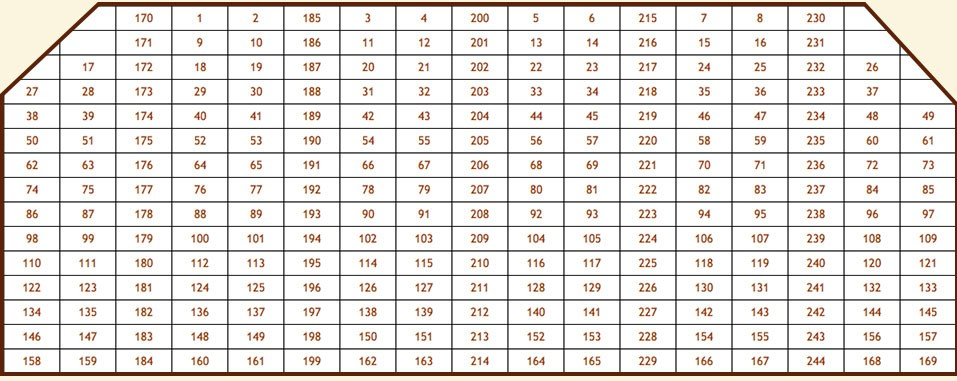## Section 8: plot numbers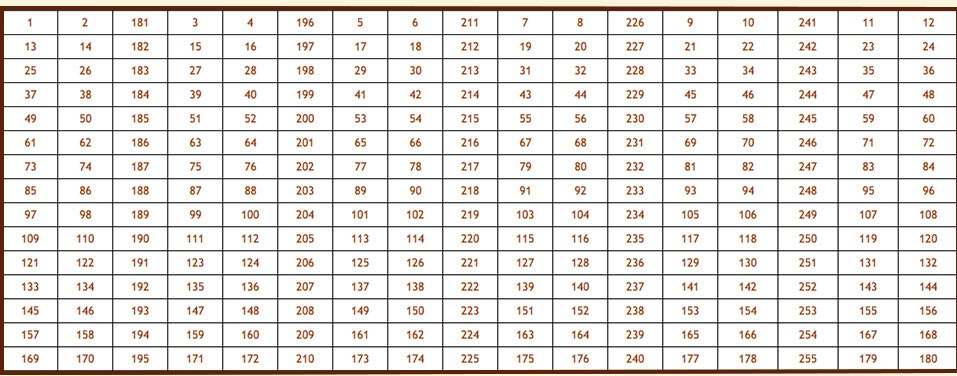## Section 9: plot numbers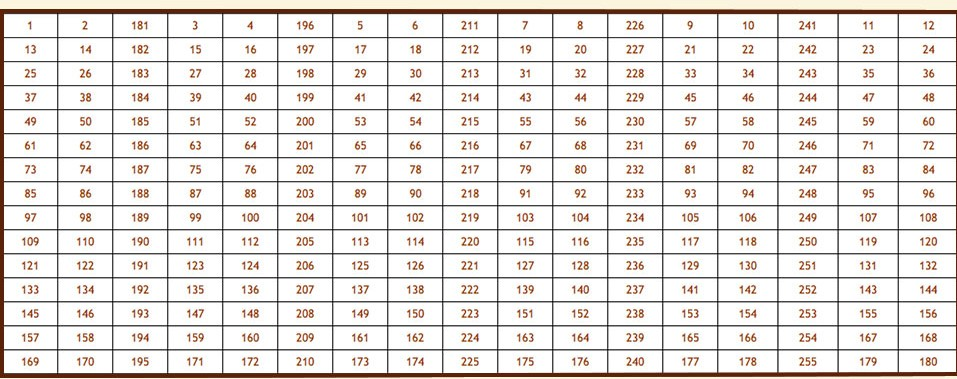## Section 10: plot numbers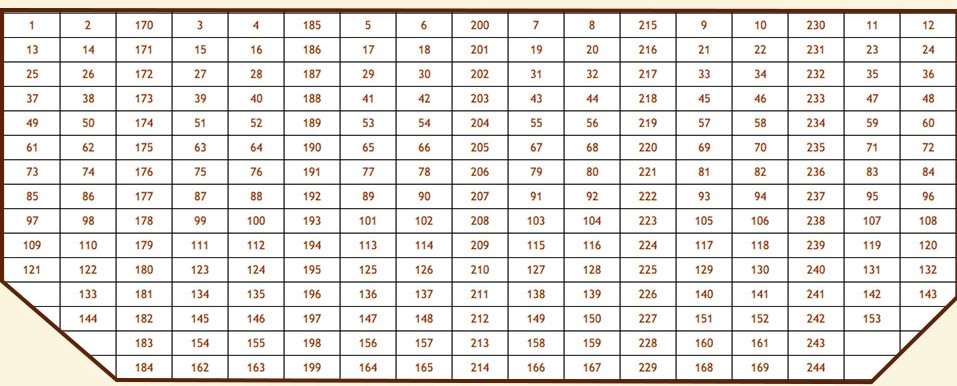## Section 11: plot numbers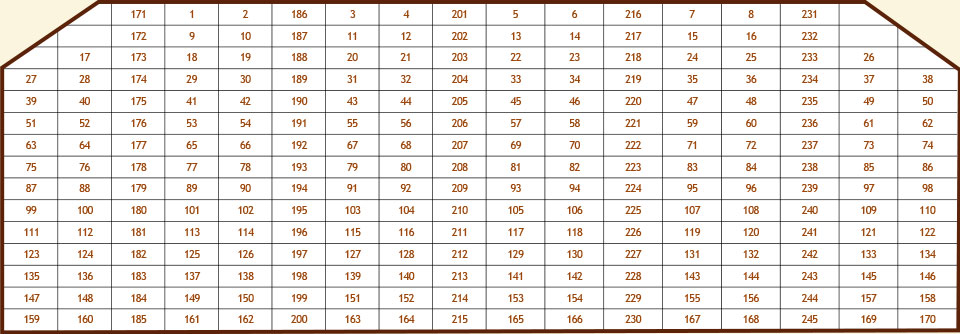## Section 12: plot numbers## Section A: plot numbers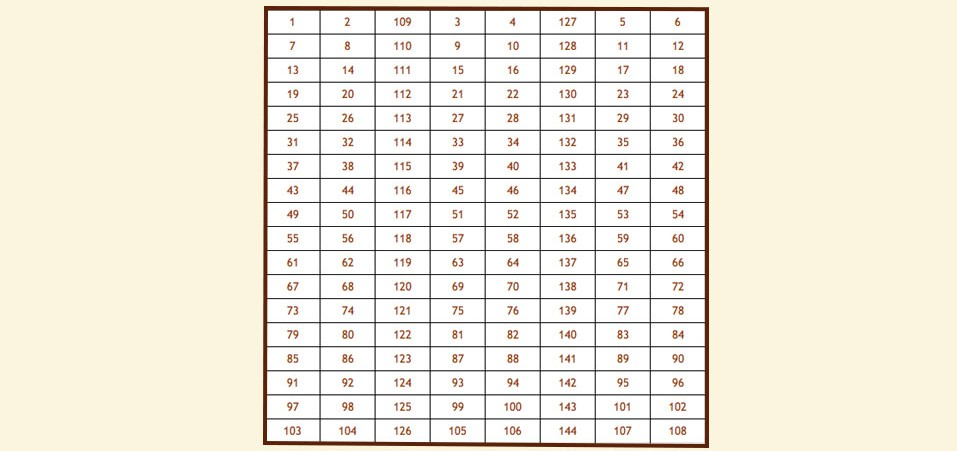## Section B: plot numbers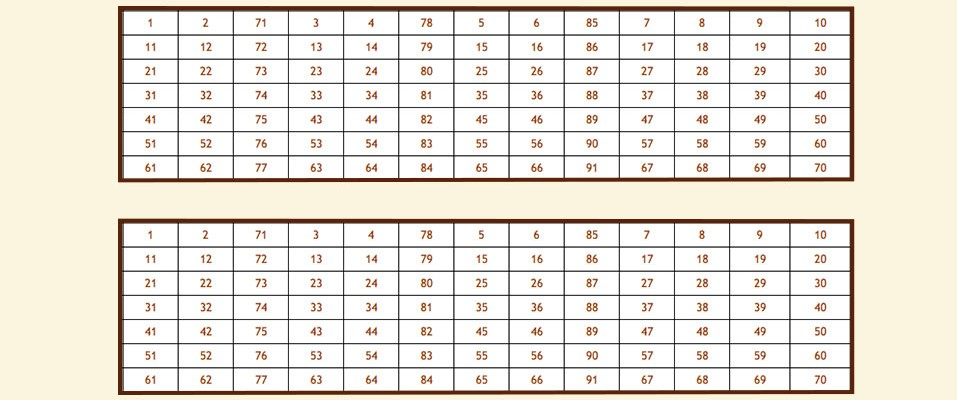## Section C: plot numbers